How Cheenta works to ensure student success?
Explore the Back-Story

# Test of Mathematics Solution Subjective 67 - Four Real Roots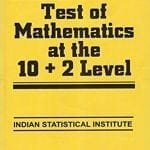This is a Test of Mathematics Solution Subjective 67 (from ISI Entrance). The book, Test of Mathematics at 10+2 Level is Published by East West Press. This problem book is indispensable for the preparation of I.S.I. B.Stat and B.Math Entrance.

Also visit: I.S.I. & C.M.I. Entrance Course of Cheenta

## Problem

Describe the set of all real numbers x which satisfy 2<1.

## Solution

2< 1

Now x< 0 is not in the domain of logarithm.

So x> 0.

Now as x>0           2x+3 > 1.

So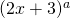>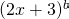for a>b
So 2< 1 or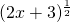> x
or 2x+3 >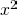or-2x -3 < 0
or -1 < x < 3

But x > 0 so set of all real number

x is 0 < x < 3.This is a Test of Mathematics Solution Subjective 67 (from ISI Entrance). The book, Test of Mathematics at 10+2 Level is Published by East West Press. This problem book is indispensable for the preparation of I.S.I. B.Stat and B.Math Entrance.

Also visit: I.S.I. & C.M.I. Entrance Course of Cheenta

## Problem

Describe the set of all real numbers x which satisfy 2<1.

## Solution

2< 1

Now x< 0 is not in the domain of logarithm.

So x> 0.

Now as x>0           2x+3 > 1.

So>for a>b
So 2< 1 or> x
or 2x+3 >or-2x -3 < 0
or -1 < x < 3

But x > 0 so set of all real number

x is 0 < x < 3.

### Knowledge Partner